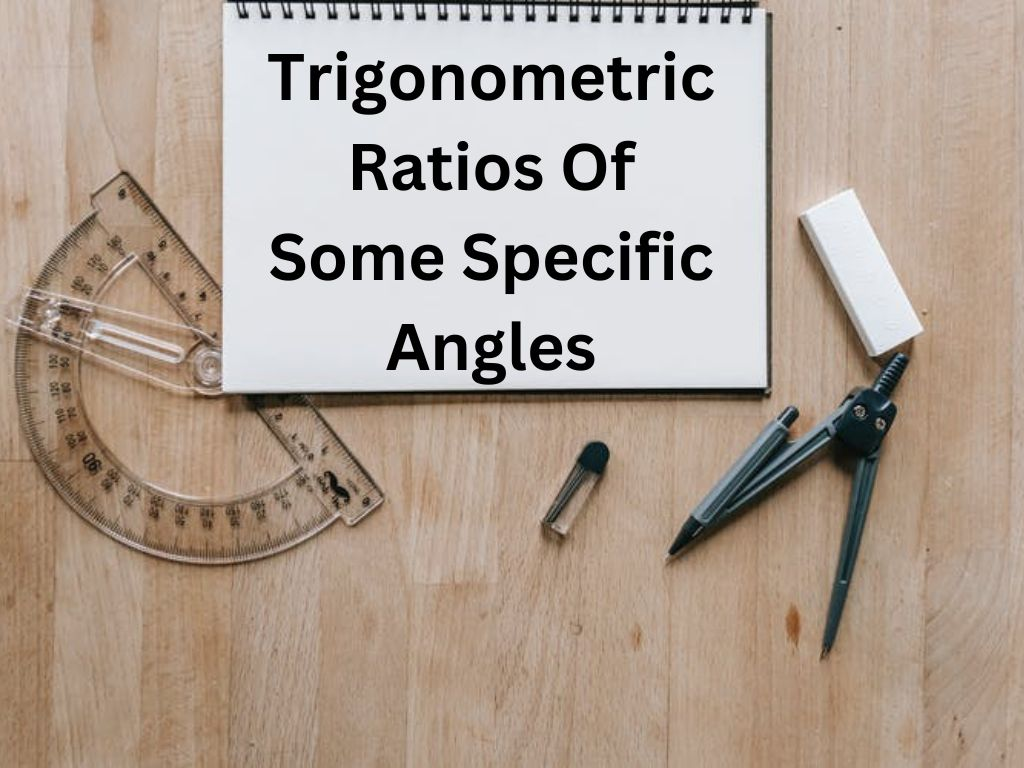## Trigonometric Ratios of Some Specific Angles Class 10th

In Trigonometry, For all the trigonometric ratios we will find their values for some specific angles 0°, 30°, 45°, 60°, and 90°. Trigonometric Ratios of Angle 0° Let △ABC be a right-angled triangle in which ∠B is the right angle and ∠C is an acute angle ϴ. If the angle ϴ becomes 0° then line …